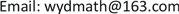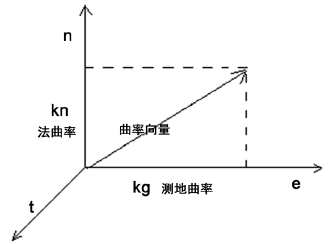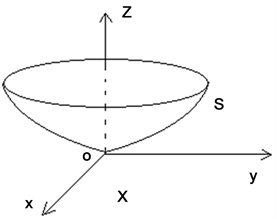﻿ 本科微分几何教学的一些探索 Some Explorations on Undergraduate Teaching of Differential Geometry

Vol. 09  No. 06 ( 2019 ), Article ID: 32813 , 5 pages
10.12677/AE.2019.96111

Some Explorations on Undergraduate Teaching of Differential Geometry

College of Mathematics and Information Science, Jiangxi Normal University, Nanchang JiangxiReceived: Oct. 12th, 2019; accepted: Oct. 28th, 2019; published: Nov. 5th, 2019ABSTRACT

In this paper, we first propose some problems on the teaching of the undergraduate course of differential geometry, and then give some advice on the teaching of the course. Our advice mainly includes: enhancing the interdisciplinary knowledge and the applications of new theory, enhancing the ability of computations and graphic method, and adding contents and hours to the course.

Keywords:Differential Geometry, Exploration of Teaching1. 引言

2. 存在的问题

1) 缺乏微分几何与其他课程的联系

2) 缺乏抽象理论与具体实践的结合

3) 计算能力和几何直观能力不足

4) 几何课程与课时显少

3. 一些教学探索

3.1. 加强知识交叉及新理论运用

$ΙΙ=Ld{u}^{2}+2Mdudv+Nd{v}^{2}=\left(du,dv\right)\left[\begin{array}{cc}L& M\\ M& N\end{array}\right]\left(\begin{array}{c}du\\ dv\end{array}\right)$

$\iint g\left(x,y\right)\text{d}x\text{d}y=\iint g\left(x\left(u,v\right),y\left(u,v\right)\right)|\frac{\partial \left(x,y\right)}{\partial \left(u,v\right)}|\text{d}u\text{d}v$

3.2. 加强实践及计算能力

3.3. 加强几何直观Figure 1. The orthogonal frame of surfaceFigure 2. The surface S and its tangent plane X

3.4. 增加课时与课程内容

Some Explorations on Undergraduate Teaching of Differential Geometry[J]. 教育进展, 2019, 09(06): 683-687. https://doi.org/10.12677/AE.2019.96111

1. 1. 梅向明, 黄敬之. 微分几何[M]. 北京: 高等教育出版社, 2003.

2. 2. 彭家贵, 陈卿. 微分几何[M]. 北京: 高等教育出版社, 2002.

3. 3. Opera, J. 微分几何及其应用[M]. 陈智奇, 李君, 译. 北京: 机械工业出版社, 2006.

4. 4. 孙和军, 赵培标, 陈大广. 微分几何的教学地位与方法[J]. 高等数学研究, 2011, 14(1): 101-103.